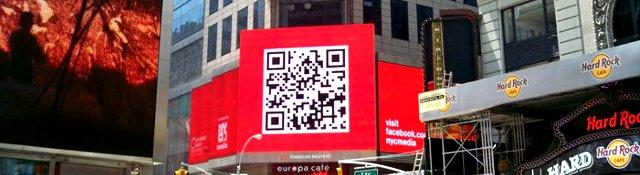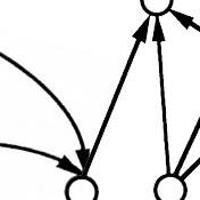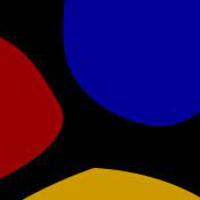## Scanning QR Codes

Recognize QR Codes in images from scratch. We'll do all the bit math to figure out the location markers and then read data from the black/white array.

## Verifying the finder patterns

Series: Scanning QR Codes:

1. Introduction
2. Locating the QR code
3. Verifying the finder patterns
4. Extracting bits from the QR code

In the previous part, we were able to locate the finder patterns based on the ratio check. In this part, we will verify if there indeed exists a finder pattern by doing multiple tests.

In the previous part, we left a command that we would revisit in the new part.

```if(currentState==4) {
if(checkRatio(stateCount)) {
// This is where we do some more checks
bool confirmed = handlePossibleCenter(img, stateCount, row, col);
} else {
currentState = 3;
...
```

Let's define this method `handlePossibleCenter` and get it to work. It's a lot of work - but is fairly easy to comprehend.

```bool qrReader::handlePossibleCenter(const Mat& img, int *stateCount, int row, int col) {
int stateCountTotal = 0;
for(int i=0;i<5;i++) {
stateCountTotal += stateCount[i];
}

// Cross check along the vertical axis
float centerCol = centerFromEnd(stateCount, col);
float centerRow = crossCheckVertical(img, row, (int)centerCol, stateCount, stateCountTotal);
if(isnan(centerRow)) {
return false;
}

// Cross check along the horizontal axis with the new center-row
centerCol = crossCheckHorizontal(img, centerRow, centerCol, stateCount, stateCountTotal);
if(isnan(centerCol)) {
return false;
}

// Cross check along the diagonal with the new center row and col
bool validPattern = crossCheckDiagonal(img, centerRow, centerCol, stateCount, stateCountTotal);
if(!validPattern) {
return false;
}
```

The function accepts the `stateCount` (as described in the previous post) and the row, column at which it found the pattern. This method first tries cross-check in the vertical axis. In the process, it computes a refined Y coordinate of the finder pattern. Next, it tries to verify the pattern along the horizontal axis (using the refined Y coordinate). In the process, it computes a refined X coordinate. Finally, it uses the refined X and Y coordinates and verifies diagonally.

If our code passes all these checks, we can be confident that this is indeed a finder pattern. However, it is likely that we may have seen it before. So the next section of code de-duplicates patterns and improves estimates at the same time.

```    Point2f ptNew(centerCol, centerRow);
float newEstimatedModuleSize = stateCountTotal / 7.0f;
bool found = false;
int idx = 0;
```

We start out by defining the newly detected finder pattern and the corresponding module size. Next, we loop through all the points and see if `ptNew` is close enough to an existing point:

```    // Definitely a finder pattern - but have we seen it before?
for(Point2f pt : possibleCenters) {
Point2f diff = pt - ptNew;
float dist = (float)sqrt(diff.dot(diff));

// If the distance between two centers is less than 10px, they're the same.
if(dist < 10) {
```

If two centers are very close by, we improve the estimate by taking their means:

```            pt = pt + ptNew;
pt.x /= 2.0f; pt.y /= 2.0f;
found = true;
break;
}
idx++;
}
```

If we didn't find it in the existing set of points, this is a fresh finder pattern. So, we push a new item to our list:

```    if(!found) {
possibleCenters.push_back(ptNew);
}

return false;
}
```

And that finishes our main checking method. We just need to implement that helper functions starting with `crossCheck` and we'll be one step closer to reading the QR code!

## Verifying in the vertical direction

The idea here is simple. If you look at the horizontal row of the red point in the image below, you can see that the ratio test would pass. However, there are two issues: - We haven't verified if the vertical direction also passes the ratio test - The red point is far from the actual center of the finder pattern

So the check in the vertical direction traverses the image in the vertical direction and works on these two short-comings. It verifies that the ratio test actually passes and also updates the center estimate to the green point.

Before we get started, I also created a new `#define` for returning `NaN` values. These will be used to indicate that the test failed and the `find` method should move on to find more patterns. Since this is quite verbose, the `#define` will help quite a bit.

```#define nan std::numeric_limits<float>::quiet_NaN();
```

With that out of the way, let's start the actual vertical check:

```float qrReader::crossCheckVertical(const Mat& img, int startRow, int centerCol, int centralCount, int stateCountTotal) {
int maxRows = img.rows;
int crossCheckStateCount = {0};
int row = startRow;
while(row>=0 && img.at<uchar>(row, centerCol)<128) {
crossCheckStateCount++;
row--;
}
if(row<0) {
return nan;
}
```

Here, we start at the provided coordinates `startRow` and `centerCol` and traverse upwards. You'll notice that we have a much simpler state logic than the `find` method. If we reach the upper boundary of the image, we simply return `nan`.

```    while(row>=0 && img.at<uchar>(row, centerCol)>=128 && crossCheckStateCount<centralCount) {
crossCheckStateCount++;
row--;
}
if(row<0 || crossCheckStateCount>=centralCount) {
return nan;
}

while(row>=0 && img.at<uchar>(row, centerCol)<128 && crossCheckStateCount<centralCount) {
crossCheckStateCount++;
row--;
}
if(row<0 || crossCheckStateCount>=centralCount) {
return nan;
}
```

These two while loops above continue the traversal in the upwards direction and keep track of the number of pixels that are white and black respectively. As before, we return `nan` if we hit the upper boundary of the image prematurely. However, we have an additional constraint now - if the dimensions of one of the outer squares is more than the central square, we return a `nan` as well.

```    // Now we traverse down the center
row = startRow+1;
while(row<maxRows && img.at<uchar>(row, centerCol)<128) {
crossCheckStateCount++;
row++;
}
if(row==maxRows) {
return nan;
}

while(row<maxRows && img.at<uchar>(row, centerCol)>=128 && crossCheckStateCount<centralCount) {
crossCheckStateCount++;
row++;
}
if(row==maxRows || crossCheckStateCount>=stateCountTotal) {
return nan;
}

while(row<maxRows && img.at<uchar>(row, centerCol)<128 && crossCheckStateCount<centralCount) {
crossCheckStateCount++;
row++;
}
if(row==maxRows || crossCheckStateCount>=centralCount) {
return nan;
}
```

The three loops above do the exact same task - but traverse downwards from the center. This is the exact same code but in the opposite direction. The `if` conditions now check if the traversal hit the lower boundary of the image.

```    int crossCheckStateCountTotal = 0;
for(int i=0;i<5;i++) {
crossCheckStateCountTotal += crossCheckStateCount[i];
}

if(5*abs(crossCheckStateCountTotal-stateCountTotal) >= 2*stateCountTotal) {
return nan;
}

float center = centerFromEnd(crossCheckStateCount, row);
return checkRatio(crossCheckStateCount)?center:nan;
}
```

Finally, we verify if the cross-check state count total is similar to the original state count total. If it is, we compute the new center, check the ratio of the cross-check state count and return an appropriate value. If the ratio is 1:1:3:1:1, we return the refined center. If not, we return `nan`.

### Verifying in the horizontal direction

The exact same idea here as the previous section - but in the horizontal direction. Only difference now is, we traverse along the green line and refine the X coordinate of the image. We will end up with the orange point.

```float qrReader::crossCheckHorizontal(const Mat& img, int centerRow, int startCol, int centerCount, int stateCountTotal) {
int maxCols = img.cols;
int stateCount = {0};

int col = startCol;
const uchar* ptr = img.ptr<uchar>(centerRow);
while(col>=0 && ptr[col]<128) {
stateCount++;
col--;
}
if(col<0) {
return nan;
}

while(col>=0 && ptr[col]>=128 && stateCount<centerCount) {
stateCount++;
col--;
}
if(col<0 || stateCount==centerCount) {
return nan;
}

while(col>=0 && ptr[col]<128 && stateCount<centerCount) {
stateCount++;
col--;
}
if(col<0 || stateCount==centerCount) {
return nan;
}
```

Code until here was traversal in the left direction. Now, we traverse right from the green center point:

```    col = startCol + 1;
while(col<maxCols && ptr[col]<128) {
stateCount++;
col++;
}
if(col==maxCols) {
return nan;
}

while(col<maxCols && ptr[col]>=128 && stateCount<centerCount) {
stateCount++;
col++;
}
if(col==maxCols || stateCount==centerCount) {
return nan;
}

while(col<maxCols && ptr[col]<128 && stateCount<centerCount) {
stateCount++;
col++;
}
if(col==maxCols || stateCount==centerCount) {
return nan;
}
```

Finally, verify if the state counts make sense return a value appropriately:

```    int newStateCountTotal = 0;
for(int i=0;i<5;i++) {
newStateCountTotal += stateCount[i];
}

if(5*abs(stateCountTotal-newStateCountTotal) >= stateCountTotal) {
return nan;
}

return checkRatio(stateCount)?centerFromEnd(stateCount, col):nan;
}
```

### Verifying in the diagonal direction

You're probably bored by now - but this is the last step! Only difference here is that we don't refine the center position anymore.

Traversal to the top-left:

```bool qrReader::crossCheckDiagonal(const Mat &img, float centerRow, float centerCol, int maxCount, int stateCountTotal) {
int stateCount = {0};

int i=0;
while(centerRow>=i && centerCol>=i && img.at<uchar>(centerRow-i, centerCol-i)<128) {
stateCount++;
i++;
}
if(centerRow<i || centerCol<i) {
return false;
}

while(centerRow>=i && centerCol>=i && img.at<uchar>(centerRow-i, centerCol-i)>=128 && stateCount<=maxCount) {
stateCount++;
i++;
}
if(centerRow<i || centerCol<i || stateCount>maxCount) {
return false;
}

while(centerRow>=i && centerCol>=i && img.at<uchar>(centerRow-i, centerCol-i)<128 && stateCount<=maxCount) {
stateCount++;
i++;
}
if(stateCount>maxCount) {
return false;
}
```

Traversal to the bottom-right from the orange center:

```    int maxCols = img.cols;
int maxRows = img.rows;
i=1;
while((centerRow+i)<maxRows && (centerCol+i)<maxCols && img.at<uchar>(centerRow+i, centerCol+i)<128) {
stateCount++;
i++;
}
if((centerRow+i)>=maxRows || (centerCol+i)>=maxCols) {
return false;
}

while((centerRow+i)<maxRows && (centerCol+i)<maxCols && img.at<uchar>(centerRow+i, centerCol+i)>=128 && stateCount<maxCount) {
stateCount++;
i++;
}
if((centerRow+i)>=maxRows || (centerCol+i)>=maxCols || stateCount>maxCount) {
return false;
}

while((centerRow+i)<maxRows && (centerCol+i)<maxCols && img.at<uchar>(centerRow+i, centerCol+i)<128 && stateCount<maxCount) {
stateCount++;
i++;
}
if((centerRow+i)>=maxRows || (centerCol+i)>=maxCols || stateCount>maxCount) {
return false;
}
```

Verify if the state count ratio is correct, etc:

```    int newStateCountTotal = 0;
for(int j=0;j<5;j++) {
newStateCountTotal += stateCount[j];
}

return (abs(stateCountTotal - newStateCountTotal) < 2*stateCountTotal) && checkRatio(stateCount);
}
```

## Drawing the detection

We've finished the hard part and we just need to render the image now! The code below is quite straight-forward. We draw rectangles at the finder patterns's center. The width and height of this rectangle is computed from the module size. Remember that the module size is equal to the width or height of one black/white tile in the QR code.

```void qrReader::drawFinders(Mat &img) {
if(possibleCenters.size()==0) {
return;
}

for(int i=0;i<possibleCenters.size();i++) {
Point2f pt = possibleCenters[i];
float diff = estimatedModuleSize[i]*3.5f;

Point2f pt1(pt.x-diff, pt.y-diff);
Point2f pt2(pt.x+diff, pt.y+diff);
rectangle(img, pt1, pt2, CV_RGB(255, 0, 0), 1);
}
}
```

## Results

Running our code should produce some really good results. Here are a few that I got:

## What's next?

We still haven't done a few important things. We haven't deciphered the contents of the QR code. This requires a bit more work and we'll start off with that in the next part.

## More in the series

This tutorial is part of a series called Scanning QR Codes:

1. Introduction
2. Locating the QR code
3. Verifying the finder patterns
4. Extracting bits from the QR code

#### Related posts#### 7 unique neural network architectures